# Physics - Classical Mechanics - Physical Pendulum

in STEMGeeks6 months ago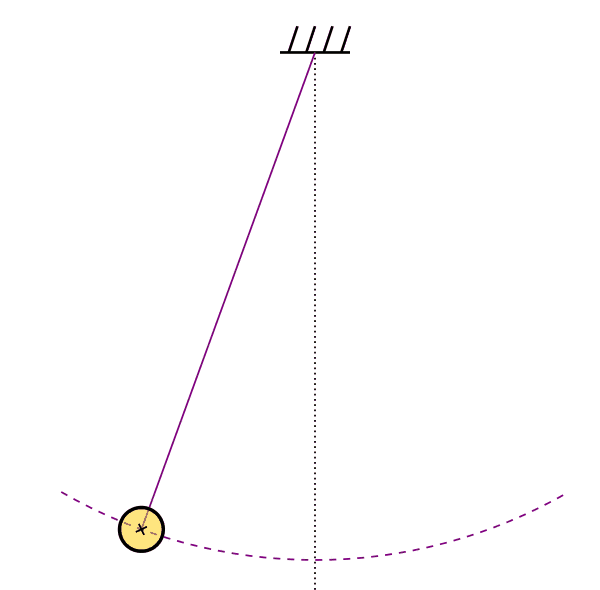[Image1]

## Introduction

Hey it's a me again @drifter1!

Today we continue with Physics, and more specifically the branch of "Classical Mechanics" in order to get into Physical Pendulums.

So, without further ado, let's get straight into it!

## Physical Pendulum

The simple pendulum that was discussed last time is an idealized model of a pendulum, where all the mass is concentrated in a single point (point mass) and the string is weight-less.

To get closer to "real" pendulums, let's now consider a rigid body that rotates about a central point without friction. When in the equilibrium position, the center of mass (or gravity - mostly they are the same) is exactly below the rotation point (an angle of ). When not at rest, we can use the angle θ in order to specify the angular position / displacement of the system, as shown below.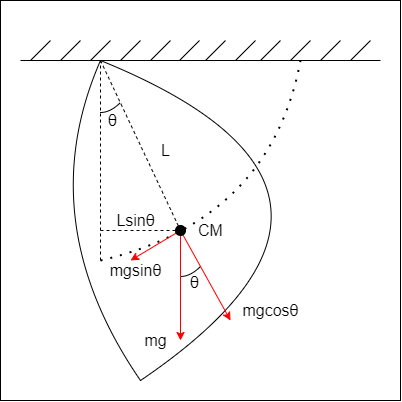Newton's laws of motion that were used last time are no longer sufficient, as angular momentum is introduced. Basically, the return force is still the force of gravity, but the torque of the corresponding gravity component has to be used instead. The return torque is given by: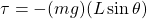The negative sign signifies that the torque "opposes" the angular displacement.

### Small Angle Approximations

Similar to the simple pendulum model, the physical pendulum is not a form of simple harmonic motion as well, because the motion is proportional to sinθ and not θ. But, for small angles we can approximate sinθ with θ, which yields: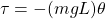Therefore, the motion equation is: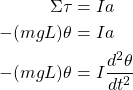where I is the the moment of inertia and a the angular acceleration (that gets replaced by the corresponding angular displacement derivative).

Solving for the angular acceleration (a), yields: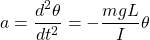and thus comparing with the corresponding equation from the fundamentals post: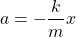we can discover that mgL / I plays a similar role to k / m, which gives us the following equations: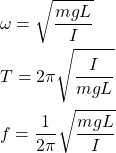The angular displacement equation is also the same: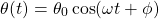### Estimating the Moment of Inertia

The physical pendulum model can be used in order to approximate the moment of inertia, by solving for I in the period equation: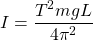## Final words | Next up

And this is actually it for today's post!

Next time we will get into exercises around Pendulums!

See ya!Keep on drifting!

Posted with STEMGeeks

Sort:Thanks for your contribution to the STEMsocial community. Feel free to join us on discord to get to know the rest of us!

Please consider delegating to the @stemsocial account (85% of the curation rewards are returned).

You may also include @stemsocial as a beneficiary of the rewards of this post to get a stronger support.